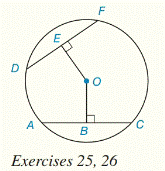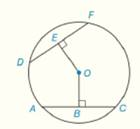Chapter 6.CR, Problem 25CRElementary Geometry For College St...

7th Edition
Alexander + 2 others
ISBN: 9781337614085

Solutions

Chapter
SectionElementary Geometry For College St...

7th Edition
Alexander + 2 others
ISBN: 9781337614085
Textbook Problem

G i v e n :                               D F - ≅ A C -   i n   ⨀ O                                                                       O E = 5 x + 4                                                                     O B = 2 x + 19 F i n d :                                             O ETo determine

To Find:

The value of OE, if DF-AC- in  O, OE=5x+4 and OB=2x+19Explanation

Approach:

Theorem 6.1.1:

A radius that is perpendicular to a chord bisects the chord.

Theorem 6.1.8:

Congruent chords are located at the same distance from the center of a circle.

Calculation:

Given that DF-AC- in O

So, DF and AC are congruent chords.

So, by Theorem 6.1.8:

Congruent chords are located at the same distance from the center of a circle.

DF and AC are at the same distance from the center of a circle  O.

So, OE=OB

But it is given that, OE=5x+4 and OB=2x+19

So, 5x+4=

Still sussing out bartleby?

Check out a sample textbook solution.

See a sample solution

The Solution to Your Study Problems

Bartleby provides explanations to thousands of textbook problems written by our experts, many with advanced degrees!

Get Started

Rewrite by completing the square. (a) x2 + x + 1 (b) 2x2 12x + 11

Single Variable Calculus: Early Transcendentals, Volume I

True or False: converges.

Study Guide for Stewart's Multivariable Calculus, 8th

For , f(x) = 0 2 3 f(3) does not exist

Study Guide for Stewart's Single Variable Calculus: Early Transcendentals, 8th